### Home > CALC > Chapter 4 > Lesson 4.3.1 > Problem4-99

4-99.

Using complete sentences, describe what each of the following graphs represents. Then, identify the physical quantity represented by the notation below the graph. Homework Help ✎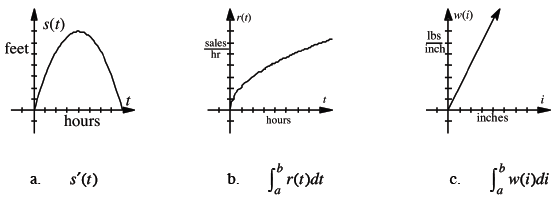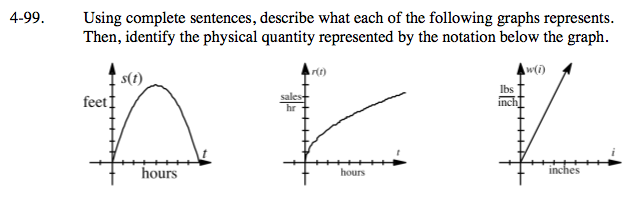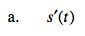$s'(t)=\frac{ds}{dt}=\text{ instantaneous }\frac{\Delta \text{feet}}{\Delta \text{time}}$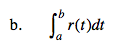$\frac{\text{sales}}{\text{hour}}$

indicates a rate graph and an integral is an area function.

Be sure to mention the significance of the bounds.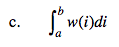Refer to the hints in part (b).